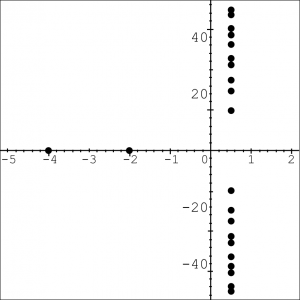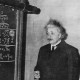# HLF Blogs: What is the Riemann Hypothesis?This week, Katie and Paul are blogging from the Heidelberg Laureate Forum – a week-long maths conference where current young researchers in maths and computer science can meet and hear talks by top-level prize-winning researchers. For more information about the HLF, visit the Heidelberg Laureate Forum website.

This year at the HLF there are multiple sessions in the program concerning the Riemann Hypothesis, including a talk from one of the laureates, and one of the young-researcher-led workshop sessions. But what exactly is the Riemann Hypothesis, and what is its place in mathematics?

## What is the Riemann Hypothesis?

The Riemann Hypothesis was conjectured in 1859 by Bernhard Riemann, a mathematician working in analysis and number theory. It concerns a function called the Riemann Zeta function, which is defined as follows:Given an ‘input’ number $s$, to calculate the value of the function, you add together the numbers $\frac{1}{1^s}$, $\frac{1}{2^s}$ and so on. For some choices of $s$, as you add more numbers the sum approaches a limit – for example if $s = 2$ the sum converges to $\frac{π^2}{6}$. But if $s$ is 1 or less, the sum diverges: it just keeps getting bigger, exceeding any possible upper bound. However, a technique known as ‘analytic continuation’ can be used to extend the function and assign finite values even in these cases. The Riemann zeta function is the version of this function extended across the entire set of numbers, including – and this is where the real interest lies – the complex numbers.

A complex number is one of the form $a + ib$, where $i$ is the square root of $-1$. Such numbers are considered to exist in a 2-dimensional plane, called the complex plane, with the “real” part a extending left-to-right like a normal number line, and another perpendicular axis extending vertically for the value of $b$ – the “imaginary” part of the number.Numbers in the complex plane can also be inputs to the Riemann Zeta function – for each point in this 2D plane you can evaluate what the function would be equal to for that input.

The thing analysts are often interested in when studying a function is where it’s equal to zero – these are points that give a nice insight into how the function behaves. For the Zeta function, there are plenty of known points that evaluate to zero, known as ‘zeroes of the function’. Infinitely many, in fact – every negative even integer will give the value zero, meaning there’s an infinite line of points spaced along the negative horizontal axis which all give zero when put through this function.

The only other points that have been found to make Zeta equal zero are on a different straight line in this diagram, called the ‘critical line’ – it’s the line where all the numbers have real part ($a$) equal to $\frac{1}{2}$, so it’s a vertical line running just to the right of the imaginary axis. The first one of these (above the horizontal, going upwards) is at $\frac{1}{2} + 14.134725\ldots i$, and they continue upwards, with identical zeroes at points below the line with negative equivalents of these values ($\frac{1}{2} – 14.134725\ldots i$ and so on).

It’s been proven that infinitely many zeroes lie on this critical line, all with real value $\frac{1}{2}$ and with no discernible pattern in their imaginary values, beyond the obvious vertical symmetry. Over 10,000,000,000,000 such zeroes have been computed numerically (initially by hand, and following work by Alan Turing in 1953, by computer), and billions of others have also been checked in the region around $\frac{1}{2} + 10^{24} i$. We also know that at least 40% of the zeroes of this function are on the critical line, and all the ones known so far are at irrational values of b – numbers that can’t be expressed as a fraction.

But what we don’t know is that there aren’t any others anywhere else – it can’t be proved that all the zeroes are either on the negative axis at even whole number points (these are know as the ‘trivial’ zeroes) or lie somewhere on the critical line. The Riemann Hypothesis is exactly this – the statement that all non-trivial zeroes of the Riemann Zeta function lie on the line $a =\frac{1}{2}$.Complex plot, showing the trivial zeroes going off to the left and the critical line on the right

People have been working on this problem for over 150 years, and as you would expect, some progress has been made towards a proof – other functions which have properties similar to the Zeta function can be studied, and a number of approaches including self-adjoint operators, random matrix theory, non-commutative geometry, statistical mechanics and even classification of quasicrystals have all been employed in attempts at a proof.

## What would the consequences of a proof be?

So what would happen if the Riemann Hypothesis was proved? The most straightforward consequence, if the least mathematically interesting, would be that the person to do it would be awarded one million dollars. The Riemann Hypothesis is one of the Millennium Prize Problems, a list of seven then unsolved questions in maths produced by the Clay Institute in 2000. To date, only one of these has been solved: the Poincaré Conjecture, by Grigori Perelman in 2003. Its inclusion on the list gives an indication of how important the result is considered among mathematicians.

The Riemann Hypothesis is closely related to the prime numbers – alternative formulations of the Zeta function involve stating it in terms of the prime numbers, and while these can’t be used to evaluate the function, it means that fully understanding the Zeta function could unlock some aspect of the as-yet-unknown patterns in the primes. Many results about primes have been proved on the condition that the Riemann Hypothesis is true. So a proof would deliver a large number of further important results ‘for free’.

Since modern encryption methods are based on prime numbers, there’s a popular idea that a proof of the Riemann Hypothesis would compromise this security – that it would literally (as it surely would figuratively) “break the internet”. An episode of the mid-2000s US TV series Numb3rs referred to this in an episode where the daughter of a mathematician who claimed to have a proof was kidnapped. Of course, the bare knowledge of the truth value of the hypothesis would have no such effect: any committed hacker would be perfectly happy with a method for compromising encryption that might be based on a false assumption, if it worked anyway. But if the techniques employed by a proof delivered a method for factorising large numbers quickly, such consequences might not be totally beyond the bounds of possibility.

Tomorrow at the HLF, mathematician and double Laureate Sir Michael Atiyah has announced he’ll be presenting “a simple proof using a radically new approach” of the Riemann Hypothesis. Such a proof would be huge news, and a big step forward for mathematics. We’re all looking forward to hearing what he has to say!

## About the authors

•#### Katie Steckles

Publicly engaging mathematician, Manchester MathsJam organiser, hairdo.
•#### Paul Taylor

Maths graduate, inveterate crossword and general puzzle doer, uses brackets excessively in writing (sorry)

### 13 Responses to “HLF Blogs: What is the Riemann Hypothesis?”

1.Paul Coombes

I am confused because the sentence “For some choices of , as you add more numbers the sum approaches a limit – for example if =2 the sum converges to π26” seems to contradict the following sentence of “But if is 1 or greater, the sum diverges: it just keeps getting bigger, exceeding any possible upper bound.” Isn’t 2 greater than 1?

•Paul Taylor

Well spotted! We’ve fixed this now.

•David Q

Yeah, it’s a typo. That sum diverges if s is less or equal to 1 (or more precisely, if the real part of s is less or equal to one).

2.John Read

This is a great explanation, thanks both. I found John Derbyshire’s book -Prime Obsession , really useful as a primer on understanding the Riemann hypothesis for those who’d like to learn more. I’m really looking forward to your report on tomorrow’s events and it must be very exciting for you both to be there. I’m sure you’ll be as surprised as the rest of us if this does lead to a ‘simple’ proof but hopefully if nothing else, some interesting new insights will be revealed.

3.David

Although Sir Michael Atiyah is one of the greatest and outstanding figures in mathematics, however I see almost no chance for someone even Sir Michael to solve this extremely huge and big crux. Most likely we are away from a solution for this unfathomable puzzle (maybe decades and even centuries). All in all if Atiyah can convince peers and experts, he will be unreachable and will receive a GOD-like character for sure, I hope so.

4.Shecky R

S’posedly Atiyah has a “simple proof” which is hard to believe (but of course “simple” is relative) and I have to wonder if it will somehow relate to the association with quasicrystals (and more generally to physics) that has been bandied about in recent years as a new approach to RH. One recent article:

https://www.iflscience.com/editors-blog/how-an-impossible-crystal-has-shed-new-light-on-a-milliondollar-math-problem/all/

5.Tumaini Chacha
6.Orfeas